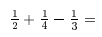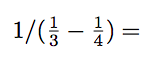February 4-22 Math Contest
First Name *
Last Name *
School *
Classroom Teacher's Name *
The sum of three numbers is 17. The first is 2 times the second. The third is 5 more than the second. What is the value of the largest of the three numbers?
1 point
Simplify. Write your answer as a fraction in simplest form (i.e. 2/3).
1 pointSimplify. Write your answer as a fraction in simplest form (i.e. 2/3).
1 pointDick is 6 years older than Jane. Six years ago he was twice as old as she was. How old is Jane now?
1 point
5 is 20% of what number?
1 point
A frog is at the bottom of a well 20 feet deep. It climbs up 3 feet everyday, but slides back 2 feet each night. If it started climbing on February 1, on what date does it get to the top of the well?
1 point
Let a = 11/15, b = 13/19, and c = 16/23. Write the letters a, b, and c in increasing order (smallest to largest).
1 point
What's the only prime integer between 90 and 100?
1 point
What is the average of 3 and 1/3? Write your answer as an improper fraction.
1 point
A right triangle has hypotenuse 29 and one leg 21. What is the length of the other leg?
1 point